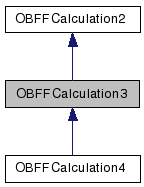# OBFFCalculation3 Class Reference

Internal class for OBForceField to hold energy and gradient calculations on specific force fields. More...

`#include <openbabel/forcefield.h>`

Inheritance diagram for OBFFCalculation3:[legend]

List of all members.

## Public Member Functions

virtual ~OBFFCalculation3 ()
virtual void SetupPointers ()

## Public Attributes

OBAtomc
int idx_c
double * pos_c
double force_c 
double energy
OBAtoma
OBAtomb
int idx_a
int idx_b
double * pos_a
double * pos_b
double force_a 
double force_b 

## Detailed Description

Internal class for OBForceField to hold energy and gradient calculations on specific force fields.

## Constructor & Destructor Documentation

 virtual ~OBFFCalculation3 ( ) ` [inline, virtual]`

Destructor.

## Member Function Documentation

 virtual void SetupPointers ( ) ` [inline, virtual]`

Returns:
Setup pointers to atom positions and forces (To be called while setting up calculations). Sets optimized to true.

Reimplemented from OBFFCalculation2.

Reimplemented in OBFFCalculation4.

## Member Data Documentation

 OBAtom* c

Used to store the atoms for this OBFFCalculation.

 int idx_c

Used to store the index of atoms for this OBFFCalculation.

 double* pos_c

Pointer to atom coordinates as double.

 double force_c

Pointer to atom forces.

 double energy` [inherited]`

Used to store the energy for this OBFFCalculation.

 OBAtom* a` [inherited]`

Used to store the atoms for this OBFFCalculation.

 OBAtom * b` [inherited]`

 int idx_a` [inherited]`

Used to store the index of atoms for this OBFFCalculation.

 int idx_b` [inherited]`

 double* pos_a` [inherited]`

Pointer to atom coordinates as double.

 double * pos_b` [inherited]`

 double force_a` [inherited]`

Pointer to atom forces.

 double force_b` [inherited]`

The documentation for this class was generated from the following file: